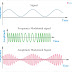The main difference between AM (Amplitude Modulation and FM (Frequency modulation) is, in Amplitude Modulation, the amplitude of a carrier wave or signal is modified but in Frequency Modulation, the frequency of the carrier wave is modified. Another important difference between AM and FM is, in Amplitude Modulation, the signal is modified according to the data which is to be transmitted while in Frequency modulation, the signal is modified as per the transmission of data.

Important differences between FM and AM are given below,

## AM VS FM

 SLNO. Amplitude Modulation(AM) Frequency Modulation(FM) 1. In Amplitude Modulation, the amplitude of the signal is varied which means the overall strength or voltage of the signal is varied. In Frequency modulation, the frequency of the signal is varied which means the no. of times of changes of direction of the current per second are varied. 2. In AM modulation the frequency and phases are kept constant. In FM modulation the amplitude and phases are kept constant. 3. In AM modulation the modulated signal uses a lower carrier frequency. In FM modulation the modulated signal uses medium frequency to very high frequency. 4. As the AM-modulated signal uses a lower frequency so it is suitable for very large-distance communication. As the FM-modulated signal uses very high frequency so it is not suitable for large-distance communication as compared to the AM-modulated signal. 5. AM modulated signal has a lower channel or has a very lower bandwidth which is why it carries less amount of data. FM-modulated signal has higher bandwidth which is why it can carry a huge amount of data. 6. The modulation index in the amplitude modulation system varies between 0 to 1 The modulation index frequency modulation system is always greater than 1 7. Amplitude Modulation has only two sidebands. Frequency modulation has an infinite number of sidebands. 8. In Amplitude Modulation, more noise occurs In Frequency Modulation less noise occurs in fact sometimes there is no noise. If there is some noise, then it can be easily removed. 9. In the Amplitude Modulation system, the circuits of the sender and receiver are very simple and have fewer components. In a Frequency modulation system, the circuits of the sender and receiver are very complex and have more components than AM modulation circuits. 10. Amplitude Modulation circuits are not so much costlier. Frequency Modulation Circuits are costlier than AM modulation circuits. 11. There is very much power wastage occurs in the amplitude modulation process. The power wastage in the frequency modulation system is very less.

## Other Differences between amplitude Modulation and Frequency Modulation

1. In Amplitude Modulation, the signal has higher wavelengths but in Frequency Modulation, the signal has lower wavelengths.

2. In amplitude modulation, the length of antennas should be large, the length may be 100 meters, but in frequency modulation, smaller length antennas required up to 1 meter

3. Amplitude Modulation most of the time affected by electromagnetic interference, but there is very less electromagnetic interference in frequency modulation.

4. The frequency range of Amplitude Modulated radios vary from 535 to 1705 KHz while the frequency range of Frequency Modulated Radios varies from 88 to 108 MHz.

5. Also, remember that amplitude modulation (AM) is typically used in one-way communication systems whereas the frequency modulation (FM) can be used in two-way radio communication system.

[Main] Differences Between AM and FM in CommunicationReviewed by Author on May 07, 2019 Rating: 5